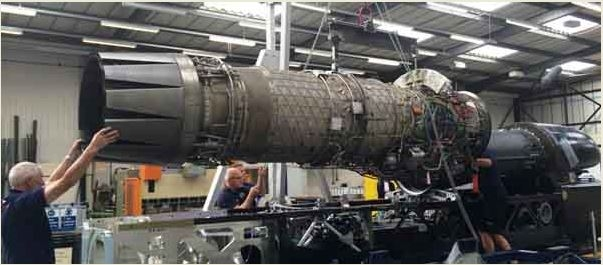# Lifting the Jet Engine into place using The Weston Differential Chain Pulley System

Education NewsThursday, 23 October, 2014

The BLOODHOUND SSC engineers are test fitting the EJ 200 to the car’s chassis ready for connection of all the engine’s services and alignment checks. The engine weighs 991 Kg and comes with a special lifting rig to ensure the engine is lifted safely and is kept level.

The chain hoist used works on the principle of the Weston Differential Pulley system and uses chains rather than rope. It has a locking system to prevent the load being accidentally dropped. This has been missed off the diagram. The system makes lifting heavy weights relatively easy.### Questions:-

1. Calculate the velocity ratio of this system over one revolution of Pulley A/B.
2. The A/B pulley combination has radii of 250 and 200mm connected to the engine giving a load of 9.71kN. Assume that the system has a typical efficiency of 40%. Calculate the effort required to lift the engine.

Note: Velocity ratio formula is the distance moved by the effort divided by the distance moved by the load.

### The solutions are below:

1. Velocity ratio formula is the distance moved by the effort divided by the distance moved by the load.

Pulley A  rotates through one revolution.

Therefore the effort will cause a length of 2πR of chain to be taken up by the right hand side of the pulley A and 2πR of chain to be let out from the left side of pulley B.

So the chain will be taken up by 2π (R-r) and the load will move a distance equal to half this value.

Velocity ratio = 2πR / π (R-r)  =  2R / (R-r)

2. Velocity Ratio = 2R / (R-r) (2 x 250) / (250-200)  = 500 / 50  = 10

η (efficiency) is Mechanical Advantage/ divided by Velocity Ratio

η = (Load / Effort) / VR)

or Effort = Load  /  (η  x  VR)

So, Effort =  9710 / (0.40 x 10)  = 242 N

An effort of 242 Newtons is required

The operator will still have to do some work to lift this engine!